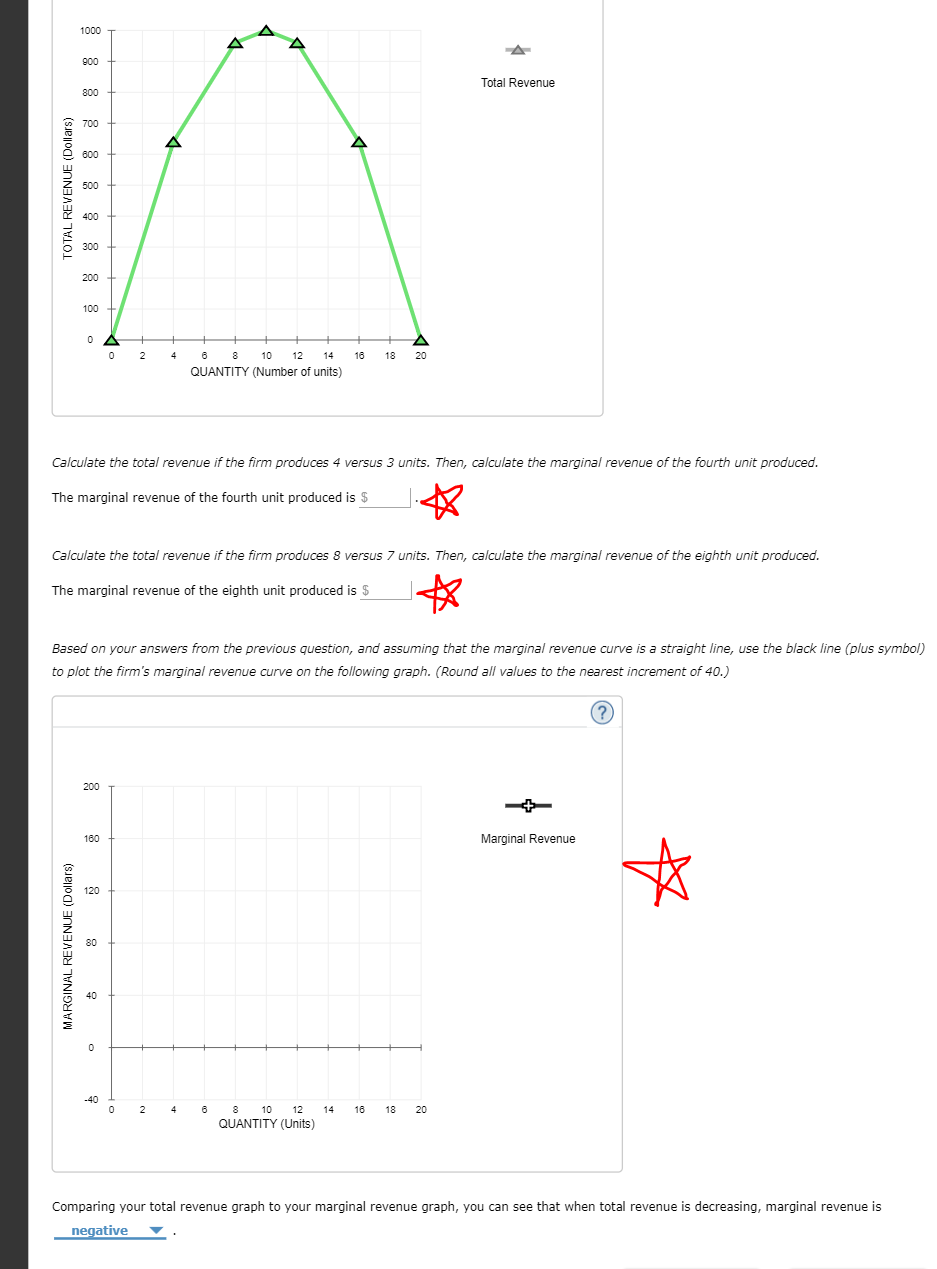Total Revenue700L 600Z 500O 3000 2 8 10 12 14 118 20QUANTITY (Number of units)Calculate the total revenue if the firm produces 4 versus 3 units. Then, calculate the marginal revenue of the fourth unit producedThe marginal revenue of the fourth unit produced is \$Calculate the total revenue if the firm produces 8 versus 7 units. Then, calculate the marginal revenue of the eighth unit producedThe marginal revenue of the eighth unit produced is \$Based on your answers from the previous question, and assuming that the marginal revenue curve is a straight line, use the black line (plus symbol)to plot the firm's marginal revenue curve on the following graph. (Round all values to the nearest increment of 40.)Marginal Revenue1200 2 810 12 141 18 20QUANTITY (Units)Comparing your total revenue graph to your marginal revenue graph, you can see that when total revenue is decreasing, marginal revenue isnegative ▼

Question

Need help with calculating the total revenue and graphing. It's the questions with a red star next to them. Thanks in advance!help_outlineImage TranscriptioncloseTotal Revenue 700 L 600 Z 500 O 300 0 2 8 10 12 14 118 20 QUANTITY (Number of units) Calculate the total revenue if the firm produces 4 versus 3 units. Then, calculate the marginal revenue of the fourth unit produced The marginal revenue of the fourth unit produced is \$ Calculate the total revenue if the firm produces 8 versus 7 units. Then, calculate the marginal revenue of the eighth unit produced The marginal revenue of the eighth unit produced is \$ Based on your answers from the previous question, and assuming that the marginal revenue curve is a straight line, use the black line (plus symbol) to plot the firm's marginal revenue curve on the following graph. (Round all values to the nearest increment of 40.) Marginal Revenue 120 0 2 810 12 141 18 20 QUANTITY (Units) Comparing your total revenue graph to your marginal revenue graph, you can see that when total revenue is decreasing, marginal revenue is negative ▼ fullscreen
Step 1

The graph you\'ve posted will probably give you total revenues figures more accurately if you just hover the cursor over the graph for each unit value. I have, however, made a table as per my estimation. This should help you approach the question if the table values don\'t match.

Step 2

Marginal revenue is the additional revenue earned for every additional unit. It can be calculated by taking the difference of 2 consecutive total revenues ( for 2 consecutive units).  Marginal Revenue for the 4th unit and the eight unit is 200 and 100 repectively as can be seen from the table.

Now, the char...

Want to see the full answer?

See Solution

Want to see this answer and more?

Our solutions are written by experts, many with advanced degrees, and available 24/7

See Solution
Tagged in